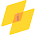## Search This Blog

### MATLAB PROGRAM:

clc ;
close all;
clear all

b=input('enter numerator coefficient of analog filter:')
a=input('enter denominator coefficient of analog filter:')
f=input('enter sampling frequency:')
Ts=1/f
[bz,az]=bilinear(b,a,f)
disp('equavilent digital transfer function H(Z)is =')
systf=tf(bz,az,Ts)

figure(1)
freqz(bz,az)
figure(2)
zplane(bz,az)

disp('the residues, pole locations and the direct terms are:') ;
[R,p,c]=residuez(bz,az)

### OUTPUT:

enter numerator coefficient of analog filter:2
b =
2
enter denominator coefficient of analog filter:[1 3 2]
a =
1     3     2
enter sampling frequency:1
f =
1

Ts =
1

bz =
0.1667    0.3333    0.1667

az =
1.0000   -0.3333    0.0000
equavilent digital transfer function H(Z)is =
systf =

0.1667 z^2 + 0.3333 z + 0.1667
------------------------------
z^2 - 0.3333 z + 7.401e-17

Sample time: 1 seconds
Discrete-time transfer function.
the residues, pole locations and the direct terms are:
R =
1.0e+15 *
0.0000
-2.2518

p =
0.3333
0.0000

c =
2.2518e+15

Subscribe:

1.verythankfull to u sir who have published it

1.Welcome..

2.Hi buddies, it is great written piece entirely defined, continue the good work constantly. water filter technologies

MATLAB